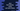# How to find the velocity in Java using user-give values## How to find the velocity in Java using user-give values:

In this post, we will learn how to find the velocity by using user provided values. There are two formulae available to calculate the velocity. The program will work for both.

### How to calculate velocity:

We can calculate velocity by using any of these two formulae:

``````velocity = u + a * t
velocity ^ 2 = u ^ 2 + 2 * a * s``````

Where,

• u is the initial velocity. Its value should be in meter/seconds.
• a is the acceleration. It should be in meter/seconds^2.
• s is the displacement. It should be in meter.
• t is the time taken for the displacement. It should be in seconds.

The program will take these values as inputs from the user and print the final velocity. u and a are common values for both. The program will ask the user either time or distance to enter and it will calculate it based on the entered value.

Let me show you the complete program:

## Java program to calculate velocity:

Below is the complete program:

``````import java.util.Scanner;

class Main {

public static void main(String[] args) {
float velocity, u, a, t, s;
Scanner sc = new Scanner(System.in);

System.out.println("Enter the initial velocity in m/s: ");
u = sc.nextFloat();

System.out.println("Enter the acceleration in m/s^2: ");
a = sc.nextFloat();

System.out.println("Enter 1 to enter time, any other value to enter displacement: ");

if (sc.nextInt() == 1) {
System.out.println("Enter time in seconds: ");
t = sc.nextFloat();
velocity = u + a * t;
} else {
System.out.println("Enter the displacement in meter: ");
s = sc.nextFloat();
velocity = (float) Math.sqrt(u * u + 2 * a * s);
}

System.out.println("Calculated velocity: "+velocity);

}
}``````

Here,

• velocity, u, a, t and s are float variables to hold the velocity, initial velocity, acceleration, time and displacement respectively.
• sc is a Scanner variable to read user inputs.
• It reads the values of u and a entered by the user.
• If user enters 1, it enters to the if block and calculates the velocity using the first formula. For any other value, it enters the else block and calculates the velocity using the second formula.
• The last line is printing the final calculated velocity.

### Sample output:

This program will give output as like below:

``````Enter the initial velocity in m/s:
0
Enter the acceleration in m/s^2:
10
Enter 1 to enter time, any other value to enter displacement:
1
Enter time in seconds:
100
Calculated velocity: 1000.0

Enter the initial velocity in m/s:
10
Enter the acceleration in m/s^2:
20
Enter 1 to enter time, any other value to enter displacement:
2
Enter the displacement in meter:
234.56
Calculated velocity: 97.37762``````

The first example uses time to calculate the velocity and the second example uses displacement to calculate it.